Most accountants treat the acquisition of an asset and the task of acquiring funds to pay for the acquisition as separate and unrelated events.

The GAAP departs from that convention only in terms of interest incurred while the asset is under construction, excluding interest incurred during its useful life. That is, interest incurred in preparing the asset for use is regarded as a cost of the asset.

Applicability

The capitalization of interest applies to non-inventory assets produced where three factors are present.

First, the firm must have incurred actual interest charges during the period, either on debt specifically obtained for the project or on debt that could have been retired if the funds used in the project had been applied for that purpose.

Second, the firm’s expenditures on the project must have involved payments of cash, exchanges of other assets, or the creation of interest-bearing debt.

In other words, the firm must have made commitments of resources that could have been avoided or redirected into retirement of debt such that interest charges could have been reduced.

Third, these expenditures must have been made while the asset was undergoing preparation for use, including activities such as planning, obtaining government permits, and actual construction.

This requirement forbids capitalization of interest during extended periods of inactivity when nothing is being done to prepare the asset for use.

Expenditure Base

The amount of interest that can be capitalized is found by applying appropriate interest rates to the average amount of accumulated expenditures.

To calculate this average amount (known as the expenditure base), the firm begins with the cost of the asset and deducts from that amount any expenditures that were made in the form of accrued liabilities or other non-interest-bearing debt.

After making this calculation, the remainder represents the resources committed to the acquisition of the asset.

The next step is to work out the average commitment during the covered time period. This is determined simply by taking one-half of the sum of the beginning and ending net expenditures.

The statement does not specify whether the average should be annual, quarterly, or monthly. The approach chosen should be selected to provide a close approximation of the typical amount committed throughout the period.

This expenditure base can be divided further into two segments if interest is attributable to both specific and general debt.

That is to say, part of the expenditure base may be financed by funds acquired specifically for the project, while the remainder of the funds came from other sources unrelated to the project.

When this condition exists, the expenditure base is partitioned before applying the appropriate interest rates.

Interest Rates

If debt financing has been obtained specifically for the construction, its interest rate should be multiplied by that portion of the expenditure base derived from that debt.

If amounts in excess of those borrowed funds have been spent, their balance should be multiplied by an average interest rate from general debt obligations.

The purpose of this calculation is to determine the amount of interest that could have been avoided if the expenditures had not been made.

Example

Suppose that Sample Company begins construction of a two-year project on 3 January 20×1.

During 20×1, the company incurs a total cost of \$400,000. At the end of the year, it has recognized non-interest-bearing liabilities (such as accounts payable) for \$100,000 of that amount.

The year-end expenditure base is thus \$300,000, or \$400,000 less \$100,000. The average accumulated expenditures for 20×1 are \$150,000, or one-half of the sum of the beginning and ending totals (\$0 plus \$300,000).

Of that amount, \$110,000 was raised through specific borrowing at 13%. The remainder was financed out of nonspecific borrowing with a rate of 9%.

The amount of capitalizable interest is computed as follows: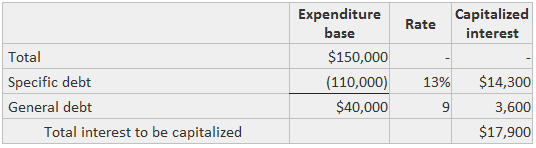If the total interest costs incurred (but unpaid) for the year are \$38,000, the following journal entry would be recorded at the end of the year: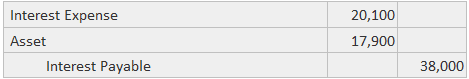Thus, \$17,900 would also be added to the expenditure base. Therefore, the balance at the beginning of 20×2 would be \$317,900.

Suppose that new expenditures of \$700,000 are incurred in 20×2. Also, suppose that all the non-interest-bearing debt is paid off and that the project is completed on 30 December. The average expenditure base would be computed as follows: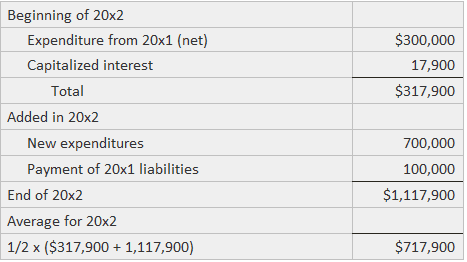Of this \$717,900, suppose that \$600,000 is financed by the 13% specific loan and the balance from the 9 percent general debt. In this case, the capitalizable interest is: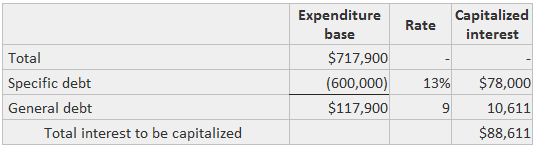If the total interest costs for the year are unpaid and equal to \$105,000, this journal entry would be recorded: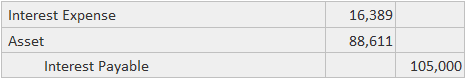The total cost of the asset can be computed as shown below: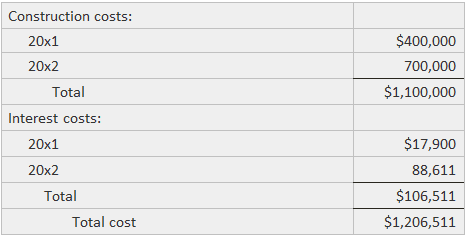The effect of capitalization is to present higher reported earnings during the period of construction. This is achieved by not expensing part of the interest cost and lowering earnings in later years through higher depreciation.

Whether these results represent a better picture or a distortion depends upon one’s judgment as to whether interest is a necessary cost of making an asset ready for use.

The following example shows a note disclosure made by Boise Cascade Corporation that is related to the capitalization of interest.